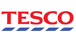Screen for the highest P/E 5y Avg Stocks in the Market

# Price to Earnings Ratio, 5 Year Average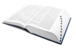What is the definition of P/E 5y Avg?

The Price to Earnings Ratio (also called the PE ratio) is the primary valuation ratio used by most equity investors. It is a measure of the price paid for a share relative to the annual net income or profit earned by the firm per share.A hig P/E ratio means that investors are paying more for each unit of net income, so the stock is more expensive compared to one with a lower P/E ratio. The P/E ratio can be seen as being expressed in years, in the sense that it shows the number of years of earnings which would be required to pay back the purchase price, ignoring inflation. Unlike the EV/EBITDA multiple which is capital structure-neutral, the price-to-earnings ratio reflects the capital structure of the company in question. The reciprocal of the P/E ratio is known as the earnings yield. For each of 5 years, we take the average share price for each year up to the annual results date, then divide by the average of the EPS for that annual result, and the previous annual result. We then calculate the average of all 5 values.Stockopedia explains P/E 5y Avg...

This is is the primary valuation ratio used by most equity investors. A high P/E ratio means that investors are paying more for each unit of net income, so the stock is more expensive compared to one with a lower P/E ratio. The P/E ratio can be seen as being expressed in years, in the sense that it shows the number of years of earnings which would be required to pay back the purchase price, ignoring inflation.
Unlike the EV/EBITDA multiple which is capital structure-neutral, the price-to-earnings ratio reflects the capital structure of the company in questionWhich Guru Screens is P/E 5y Avg used in?
Value Investing:
Growth Investing:

### The 5 lowest P/E 5y Avg Stocks in the Market

Ticker Name P/E 5y Avg StockRank
ASX:ERM Emmerson Resources 0 53
LON:CPT Concepta 0 0
CVE:GGL Ggl Resources 0 47
CVE:LBL Lattice Biologics 0 73
EPA:ALIMP Implanet SA 0 9
Screen for more high P/E 5y Avg Stocks

## Valuation Ratios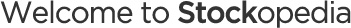Let’s get you setup so you get the most out of our serviceBrilliant - You've created a folio! Now let's add some stocks to it.
•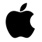Apple (AAPL)
•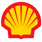Shell (RDSA)
•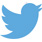•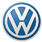•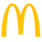•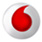•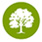•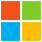•